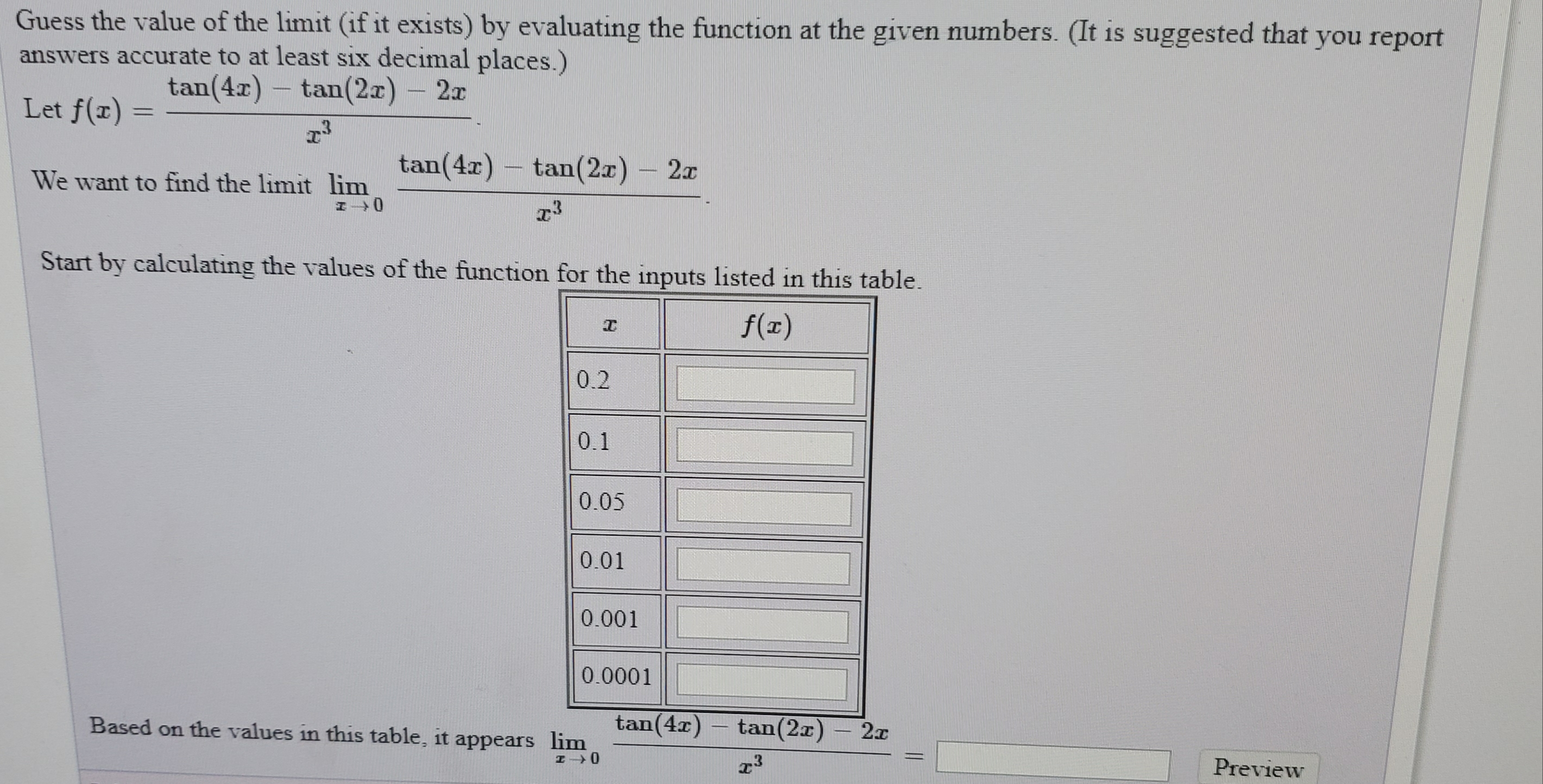# Guess the value of the limit (if it exists) by evaluating the function at the given numbers. (It is suggested that you reportanswers accurate to at least six decimal places.)tan(4r) – tan(2x)- 2xLet f(x) =tan(4x)tan(2x) - 2x–We want to find the limit limStart by calculating the values of the function for the inputs listed in this table.f(x)0.20.10.050.010.0010.0001tan(4x)tan(2x) - 2Based on the values in this table, it appears limPreview%3D

Question
10 viewshelp_outlineImage TranscriptioncloseGuess the value of the limit (if it exists) by evaluating the function at the given numbers. (It is suggested that you report answers accurate to at least six decimal places.) tan(4r) – tan(2x) - 2x Let f(x) = tan(4x) tan(2x) - 2x – We want to find the limit lim Start by calculating the values of the function for the inputs listed in this table. f(x) 0.2 0.1 0.05 0.01 0.001 0.0001 tan(4x) tan(2x) - 2 Based on the values in this table, it appears lim Preview %3D fullscreen
check_circle

Step 1

Given: find the limit of

Step 2

Now, calculating the values of function for different values of x.

 x f(x) 0.2 25.85566 0.1 20.08318 0.05 19.00290 0.01 18.67990 0.001 18.66679 0.0001 18.66666

So, form the table, we guess the value of limit of function for the value of x tends to 0, then the value of function tends to 18.666667. Hence, the value function for limit x tends to 0 is 18.666667.

Step 3

Now, we check the limit by solving the...

### Want to see the full answer?

See Solution

#### Want to see this answer and more?

Solutions are written by subject experts who are available 24/7. Questions are typically answered within 1 hour.*

See Solution
*Response times may vary by subject and question.
Tagged in

### Calculus